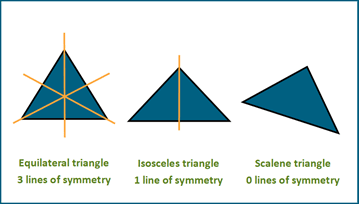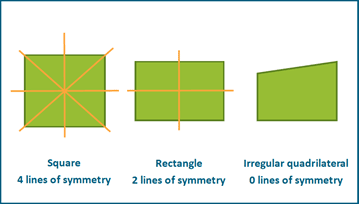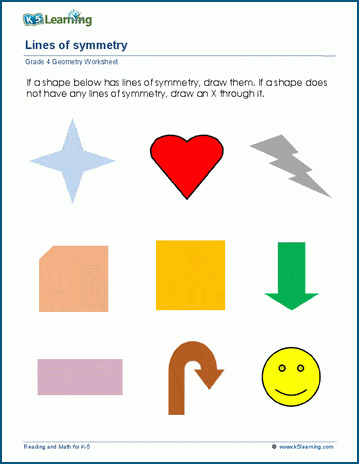# Line of Symmetry Explained

## What is a line of symmetry?

A line of symmetry is a line which divides a shape into two parts that match exactly. One way to easily work out if a shape has a line of symmetry is to fold it. When the folded part sits perfectly on top with all edges matching the fold line is a line of symmetry.

Let’s look at a few shapes and their lines of symmetry.

## Triangles line of symmetry

A triangle can have three, one or zero lines of symmetry:

An equilateral triangle has three lines of symmetry.

An isosceles triangle has one line of symmetry.

A scalene triangle has no lines of symmetry.A square has four lines of symmetry.

A rectangle has two lines of symmetry.

An irregular quadrilateral has no lines of symmetry.## Polygons line of symmetry

Polygons are shapes with all equal sides and all equal angles.

The equilateral triangle and the square are both polygons, with three and four lines of symmetry respectively.

A regular pentagon has five lines of symmetry.

A regular hexagon has six lines of symmetry.

A regular heptagon has seven lines of symmetry.

## Lines of symmetry worksheets

In our grade 4 geometry section, we have a set of worksheets for students to practice identifying and drawing lines of symmetry.